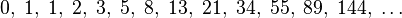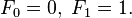# Frequently asked Java coding interview questions and answers

## Frequently asked Java coding interview questions and answers

In this post we will discuss some of the frequently asked Java coding interview questions and answers for freshers. These questions may also be asked for experience level up to 2 years. While attending a Java interview, the interviewer will not only test the understanding of basic concepts but also will test your ability to write programs. So it is important that you be thorough with the logic for some of the common problems. If you didn’t prepare for it, then it may be tough to get the logic on the spot. Need more presence of mind.

### Write a program to find if a given number is an Armstrong number.

Armstrong number is a number which is equal to sum of digits raised to the power of total number of digits in the number. Example: 371 = 3^3 + 7^3 + 1^3;

### Write a program to find if a given number is a Palindrome number.

A palindrome number is a number whose reverse is also the same. e.g. 121, 212 etc.

Another version of program using string reverse.

### Write a program to find if a given string is a Palindrome.

A string is said to be palindrome if its reverse is also same.

Another way to solve the problem.

### Write a program to find length of a string without using inbuilt functions.

In this program we are expected to find the length of the given string without using inbuilt functions like length() etc.

### Write a program to print Fibonacci series of N numbers.

In mathematics, the Fibonacci numbers or Fibonacci series or Fibonacci sequence are the numbers in the following sequence:By definition, the first two numbers in the Fibonacci series are 0 and 1, and each subsequent number is the sum of the previous two.

In mathematical terms, the sequence Fn of Fibonacci numbers is defined by the recurrence relationwith valuesNow let us see the program using recursion.

I hope that you find these list of programs useful. We will see some more programs in upcoming posts. It is important that you get accustomed with two or more logic for each program because sometimes the interviewer may ask you to solve the program without using inbuilt functions.

Also read my posts on Frequently asked Java interview questions and answers.

The following two tabs change content below.

#### Uday

Working as a Java developer since 2010. Passionate about programming in Java. I am a part time blogger.